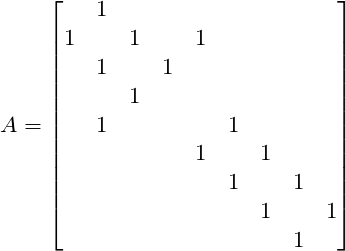# 通信题_Good IntegerC  = [3, 6, 4, 2, 5, 4, 3, 2, 1]
C' = [0, 0, 0, 0, 0, 0, 0, 0, 0]
for i in V:
for j in G[i]:
C'[j]+=C[i]

G2中的每一个点为一个数对(i,j)，其中i为G中的一个点，j为[0,Vec[i)中的一个整数。

void build_graph(){
for(int i=1;i<=9;i++){
for(auto ct:G[i]){
for(int j=0;j<vec[i];j++){
for(int k=0;k<vec[ct];k++)
if(fr[ct][k].size()<2){
G2[i][j].PB(MP(MP(ct,k),fr[ct][k].size()));
fr[ct][k].PB(MP(i,j));
break;
}
}
}
}
}

#include <bits/stdc++.h>
using namespace std;
typedef long long ll;
typedef pair<int,int> pii;
#define set0(x) memset(x,0,sizeof(x))
#define F first
#define S second
#define PB push_back
#define MP make_pair
#define rep(i, a, b) for(int i = a; i < (b); ++i)
#define trav(a, x) for(auto& a : x)
#define all(x) x.begin(), x.end()
#define sz(x) (int)(x).size()
vector<int> G;
vector< pair<pii,int> > G2;
vector< pii > fr;

int vec = {0,3,6,4,2,5,4,3,2,1,0};
void ins(int a,int b){
G[a].push_back(b);
G[b].push_back(a);
}

void build_graph(){
for(int i=1;i<=9;i++){
for(auto ct:G[i]){
for(int j=0;j<vec[i];j++){
for(int k=0;k<vec[ct];k++)
if(fr[ct][k].size()<2){
G2[i][j].PB(MP(MP(ct,k),fr[ct][k].size()));
fr[ct][k].PB(MP(i,j));
break;
}
}
}
}
}

void solve1(){
int n;string s;
cin>>n>>s;
pii pos(1,0);
string ans;
for(int i=0;i<s.size();i++){
ans+=(char)('0'+pos.F);
pos = fr[pos.F][pos.S][s[i]-'A'];
}
ans+=(char)('0'+pos.F);
reverse(all(ans));
ans+=((string)"25678").substr(0,pos.S);
cout<<ans<<endl;
}

void solve2(){
int n;string s;
cin>>n>>s;
pii pos(s-'0',(map<char,int>{{'1',0},{'2',1},{'5',2},{'6',3},{'7',4},{'8',5}}[s[s.size()-1]]));
while(s[s.size()-1]!='1')s = s.substr(0,s.size()-1);
string ans;
for(int i=1;i<s.size();i++){
for(auto ct:G2[pos.F][pos.S]){
if(ct.F.F == s[i]-'0'){
ans+=ct.S+'A';
pos = ct.F;
break;
}
}
}
reverse(all(ans));
cout<<ans<<endl;
}
string s;
int main() {
ins(1,2);ins(2,3);ins(2,5);ins(3,4);
ins(5,6);ins(6,7);ins(7,8);ins(8,9);
cin>>s;
build_graph();
if(s == "first"){
solve1();
}else{
solve2();
}
return 0;
}